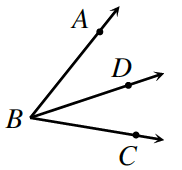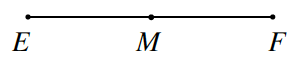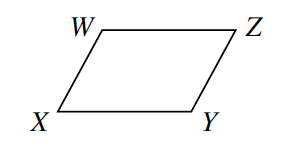### Home > CCG > Chapter Ch7 > Lesson 7.2.1 > Problem7-54

7-54.

Use the information given for each diagram below to solve for $x$. Show all work.

1. $\overrightarrow{BD}$ bisects $∠ABC$. (Remember that this means it divides the angle into two equal parts.) If $m∠ABD=5x-10°$ and $m∠ABC=65°$, solve for $x$.

$2(5x-10)=65°$2. Point $M$ is a midpoint of $\overline{EF}$. If $EM=4x-2$ and $MF=3x+9$, solve for $x$.

If $M$ is the midpoint, how are the lengths of $\overline{EM}$ and $\overline{MF}$ related?3. $WXYZ$ at right is a parallelogram. If $m∠W=9x-3°$ and $m∠Z=3x+15°$, solve for $x$.

How are the angles of the parallelogram related?